# Samacheer Kalvi 12th Computer Science Solutions Chapter 5 Python – Variables and Operators

Students can Download Computer Science Chapter 5 Python -Variables and Operators Questions and Answers, Notes Pdf, Samacheer Kalvi 12th Computer Science Book Solutions Guide Pdf helps you to revise the complete Tamilnadu State Board New Syllabus and score more marks in your examinations.

## Tamilnadu Samacheer Kalvi 12th Computer Science Solutions Chapter 5 Python – Variables and Operators

### Samacheer Kalvi 12th Computer Science Python – Variables and Operators Text Book Back Questions and Answers

PART – 1

Computer Science Chapter 5 Question 1.
Who developed Python?
(a) Ritche
(b) Guido Van Rossum
(c) Bill Gates
(d) Sunder Pitchai
(b) Guido Van Rossum

12th Computer Science Book Back Answers Question 2.
The Python prompt indicates that Interpreter is ready to accept instruction?
(a) >>>
(b) <<<
(c) #
(d) <<
(a) >>>

12th Cs Book Back Answers Question 3.
Which of the following shortcut is used to create new Python Program?
(a) Ctrl + C
(b) Ctrl + F
(c) Ctrl + B
(d) Ctrl + N
(d) Ctrl + N

12th Computer Science Book Back Answers Pdf Question 4.
Which of the following character is used to give comments in Python Program?
(a) #
(b) &
(c) @
(d) $Answer: (a) # Samacheer Kalvi Guru 12th Computer Science Question 5. This symbol is used to print more than one item on a single line? (a) Semicolon (b) Dollor($)
(c) Comma(,)
(d) Colon(;)
(c) Comma(,)

12th Computer Science Solution Book Question 6.
Which of the following is not a token?
(a) Interpreter
(b) Identifiers
(c) Keyword
(d) Operators
(a) Interpreter

12th Computer Science Samacheer Kalvi Question 7.
Which of the following is not a Keyword in Python?
(a) Break
(b) While
(c) Continue
(d) Operators
(d) Operators

Class 12 Computer Science Book Back Answers Question 8.
Which operator is also called as Comparative operator?
(a) Arithmetic
(b) Relational
(c) Logical
(d) Assignment
(b) Relational

12th Computer Book Back Answers Question 9.
Which of the following is not Logical operator?
(a) And
(b) Or
(c) Not
(d) Assignment
(d) Assignment

Samacheer Kalvi 12th Computer Science Book Back Answers Question 10.
Which operator is also called as Conditional operator?
(a) Ternary
(b) Relational
(c) Logical
(d) Assignment
(a) Ternary

PART – II

Samacheer Kalvi 12th Computer Science Question 1.
What are the different modes that can be used to test Python Program?

• In Python, programs can be written in two ways namely Interactive mode and Script mode.
• The Interactive mode allows us to write codes in Python command prompt (>>>)
• In script mode programs can be written and stored as a separate file with the extension .py and executed.
• Script mode is used to create and edit Python source files.

Tn 12th Computer Science Book Back Answers Question 2.
Write short notes on Tokens?
Python breaks each logical line into a sequence of elementary lexical components known as Tokens. The normal token types are;

1. Identifiers
2. Keywords
3. Operators
4. Delimiters
5. Literals

Whitespace separation is necessary between tokens, identifiers or keywords.

Samacheerkalvi.Guru Computer Science Question 3.
What are the different operators that can be used in Python?
The operators that can be used in Python

• Arithmetic operators
• Relational or Comparative operator
• Logical operators
• Assignment operators
• Conditional operator

Samacheer Kalvi 12th Computer Science Book Question 4.
What is a literal? Explain the types of literals?
Literal is raw data given in a variable or constant. In Python, there are various types of literals.

1. Numeric
2. String
3. Boolean

12th Computer Science Samacheer Question 5.
Write short notes on Exponent data?
An Exponent data contains decimal digit part, decimal point, exponent part followed by one or more digits.
Example: 12.E04, 24.e04.

PART – III

12 Computer Science Book Back Answers Question 1.
Write short notes on the Arithmetic operator with examples?
Arithmetic operators:
An arithmetic operator is a mathematical operator that takes two operands and performs a calculation on them. They are used for simple arithmetic. Most computer languages contain a set of such operators that can be used within equations to perform different types of sequential calculations.
Python supports the following Arithmetic operators.Samacheer Kalvi Class 12 Computer Science Question 2.
What are the assignment operators that can be used in Python?
Assignment operators:
In Python, = is a simple assignment operator to assign values to variables. Let a = 5 and b = 10 assigns the value 5 to a and 10 to b this two assignment statement can also be given as a, b = 5, 10 that assigns the value 5 and 10 on the right to the variables a and b respectively. There are various compound operators in Python like + =, – =,* =, / = % =,** = and //= are also available.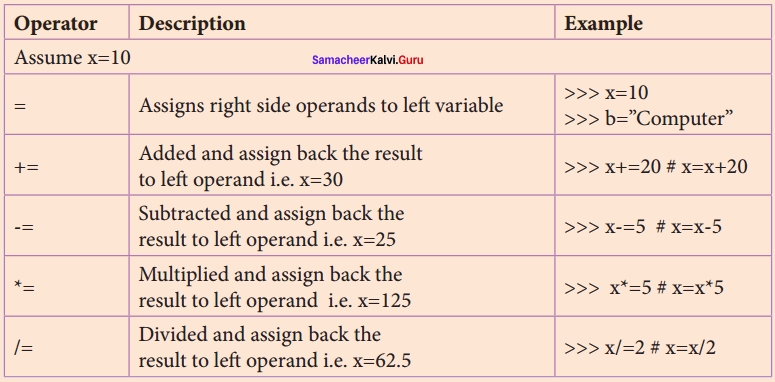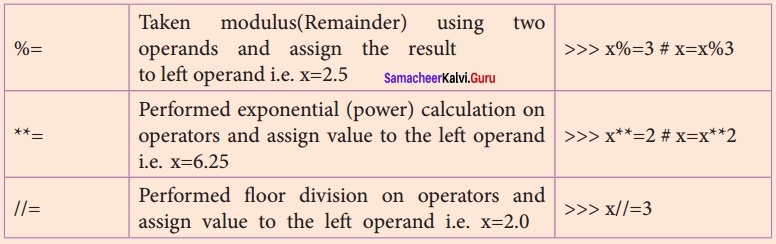12th Computer Science Chapter 7 Book Back Answers Question 3.
Explain Ternary operator with examples?

• Ternary operator is also known as conditional operator that evaluate something based on a condition being true or false.
• It simply allows testing a condition in a single line replacing the multiline if-else making the code compact.
Variable Name = [on_true] if [Test expression] else [on_false]
• Example:
min = 50 if 49 < 50 else 70 / / min = 50
min = 50 if 49 < 50 else 70 / / min = 50 Question

Question 4.
Write short notes on Escape sequences with examples?
Escape Sequences:
In Python strings, the backslash “\” is a special character, also called the “escape” character. It is used in representing certain whitespace characters: “\t” is a tab, “\n” is a newline, and “\r” is a carriage return. For example to print the message “It’s raining”, the Python command is >>> print (“It\’s rainning”)
It’s raining
Python supports the following escape sequence characters.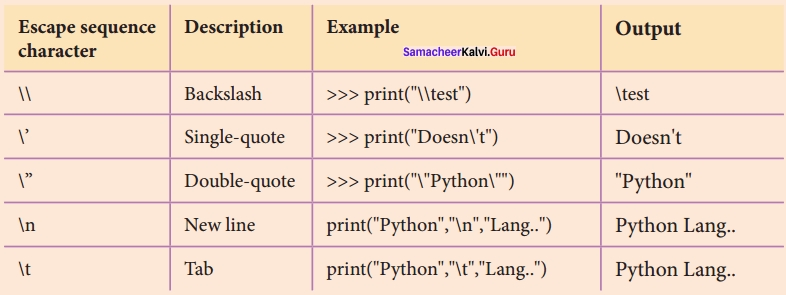12th Computer Science Book Answers Question 5.
What are string literals? Explain?

• In Python, a string literal is a sequence of 0 characters surrounded by quotes.
• Python supports Single, double, and triple quotes for a string.
• A character literal is a single character surrounded by single or double-quotes.
• The value with triple-quote u is used to give multi-line string literal.

Example:
#Demo Program to test String Literals
strings = “This is Python”
char = “C”
multiline_str = “”This is a multiline string with more than one line code.'”
print (strings)
print (char)
print (multiline_str)
# End of the Program
Output:
This is Python C
This is a multiline string with more than one line code.

PART – IV

5th Computer Science Guide Question 1.
Describe in detail the procedure Script mode programming?
Script mode Programming:
Basically, a script is a text file containing the Python statements. Python Scripts are reusable code. Once the script is created, it can be executed again and again without retyping. The Scripts are editable.
Creating Scripts in Python:
(I) Choose File ? New File or press Ctrl + N in Python shell window.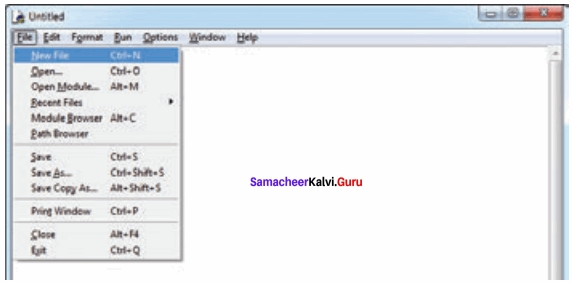(II) An untitled blank script text editor will be displayed on screen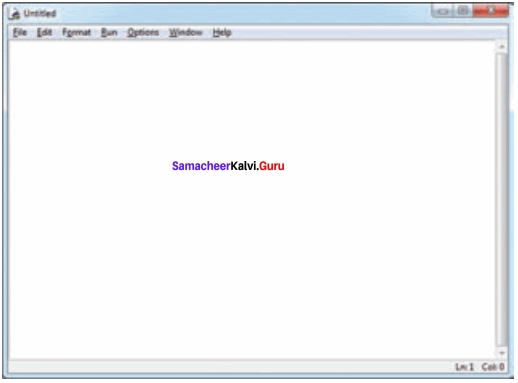(III) Type the following code in Script editor
a = 100
b = 350
c = a + b
print (“The Sum = “, c)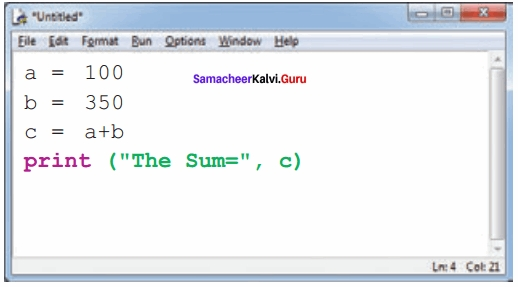Saving Python Script
(I) Choose File ? Save or Press Ctrl + S(II) Now, Save As dialog box appears on the screen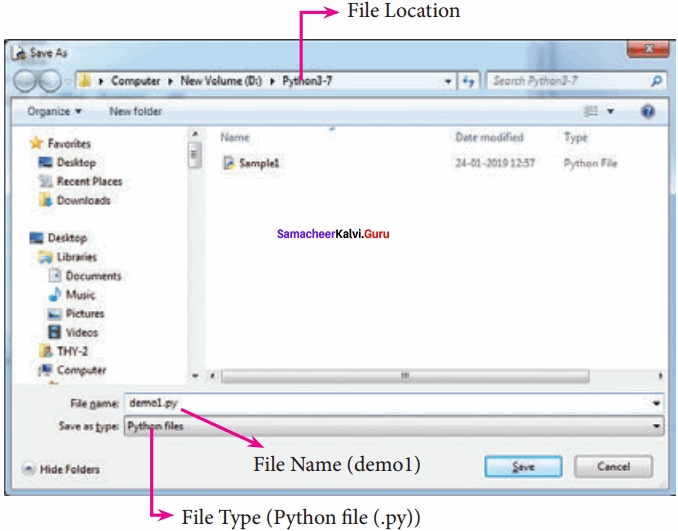(III) In the Save As dialog box, select the location where you want to save your Python code, and type the file name in the File Name box. Python files are by default saved with the extension .py. Thus, while creating Python scripts using Python Script editor, no need to specify the file extension.

(IV) Finally, click the Save button to save your Python script.
Executing Python Script

(I) Choose Run ? Run Module or Press F5(II) If your code has any error, it will be shown in red color in the IDLE window, and Python describes the type of error occurred. To correct the errors, go back to Script editor, make corrections, save the file using Ctrl + S or File ? Save and execute it again.

(III) For all error free code, the output will appear in the IDLE window of Python: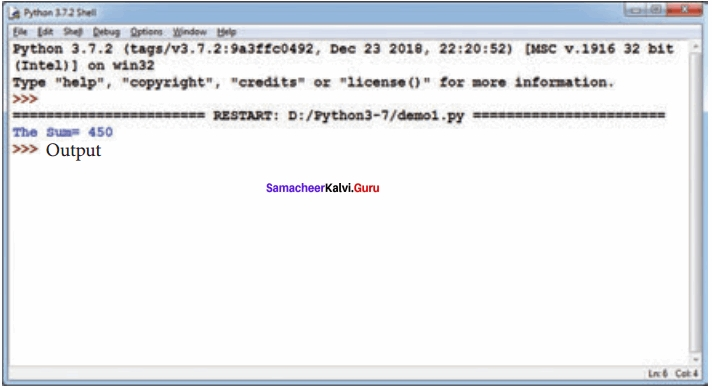Question 2.
Explain input ( ) and print ( ) functions with examples?
Input and Output Functions:
A program needs to interact with the user to accomplish the desired task; this can be achieved using Input-Output functions. The input ( ) function helps to enter data at run time by the user and the output function print ( ) is used to display the result of the program on the screen after execution.
The print ( ) function
In Python, the print Q function is used to display results on the screen. The syntax for print Q is as follows:
Example
print (“string to be displayed as output ”)
print (variable)
print (“String to be displayed as output ”, variable)
print (“String1 ”, variable, “String 2”, variable, “String 3”)
Example
>>> print (“Welcome to Python Programming”)
Welcome to Python Programming
>>> x = 5
>>> y = 6
>>> z = x + y
>>> print (z)
11
>>> print (“The sum = ”, z)
The sum = 11
>>> print (“The sum of”, x, “and”, y, “is”, z)
The sum of 5 and 6 is 11
Th print ( ) evaluates the expression before printing it on the monitor. The print ( ) displays an entire statement which is specified within print ( ). The comma ( , ) is used as a separator in print ( ) to print more than one item.
input ( ) function
In Python, input ( ) function is used to accept data as input at run time. The syntax for input ( ) function is,
Variable = input (“prompt string”)
Where, prompt string in the syntax is a statement or message to the user, to know what input can be given.
If a prompt string is used, it is displayed on the monitor; the user can provide expected data from the input device. The input ( ) takes whatever is typed from the keyboard and stores the entered data in the given variable. If prompt string is not given in input ( ) no message is displayed on the screen, thus, the user will not know what is to be typed as input.

Example 1:
input ( ) with prompt string
>>> city = input (“Enter Your City: ”)
>>> print (“I am from “, city)

Example 2:
input ( ) without prompt string
>>> city = input ( )
Rajarajan
>>> print (I am from”, city)
I am from Rajarajan
Note that in example – 2, the input ( ) is not having any prompt string, thus the user will not know what is to be typed as input. If the user inputs irrelevant data as given in the above example, then the output will be unexpected. So, to make your program more interactive, provide prompt string with input ( ).
The input ( ) accepts all data as string or characters but not as numbers. If a numerical value is entered, the input values should be explicitly converted into numeric data type. The int ( ) function is used to convert string data as integer data explicitly. We will leam about more such functions in later chapters.

Example 3:
x = int (input(“Enter Number 1: ”))
y = int (input(“Enter Number 2: ”))
print (“The sum =”, x + y)
Output:
Enter Number 1:34
Enter Number 2:56
The sum = 90

Example 4:
Alternate method for the above program
x, y = int (input(“Enter Number 1 :”)), int(input(“Enter Number 2:”))
print (”X = “,x,”Y = “,y)
Output:
Enter Number 1:30
Enter Number 2:50
X = 30 Y= 50

Question 3.
Discuss in detail Tokens in Python?
Tokens:
Python breaks each logical line into a sequence of elementary lexical components known as Tokens. The normal token types are

1. Identifiers
2. Keywords
3. Operators
4. Delimiters
5. Literals.

Whitespace separation is necessary between tokens, identifiers or keywords.

(I) Identifiers:
An Identifier is a name used to identify a variable, function, class, module or object.

1. An identifier must start with an alphabet (A..Z or a..z) or underscore ( _ ).
2. Identifiers may contain digits (0 .. 9)
3. Python identifiers are case sensitive i.e. uppercase and lowercase letters are distinct.
4. Identifiers must not be a python keyword.
5. Python does not allow punctuation character such as %, $, @ etc., within identifiers. Example of valid identifiers Sum, total _ marks, regno, num 1 Example of invalid identifiers 12 Name, name$, total – mark, continue

(II) Keywords
Keywords are special words used by Python interpreter to recognize the structure of program. As these words have specific meaning for interpreter, they cannot be used for any other purpose.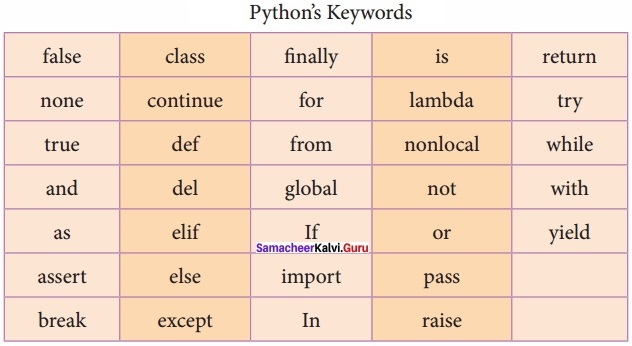(III) Operators
In computer programming languages operators are special symbols which represent computations, conditional matching etc. The value of an operator used is called operands. Operators are categorized as Arithmetic, Relational, Logical, Assignment etc. Value and variables when used with operator are known as operands.

Arithmetic operators:
An arithmetic operator is a mathematical operator that takes two operands and performs a calculation on them. They are used for simple arithmetic. Most computer languages contain a set of such operators that can be used within equations to perform different types of sequential calculations.
Python supports the following Arithmetic operators.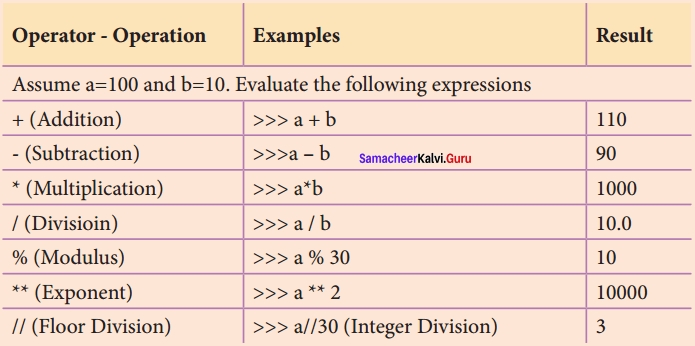Relational or Comparative operators:
A Relational operator is also called a Comparative operator which checks the relationship between two operands. If the relation is true, it returns True; otherwise, it returns False.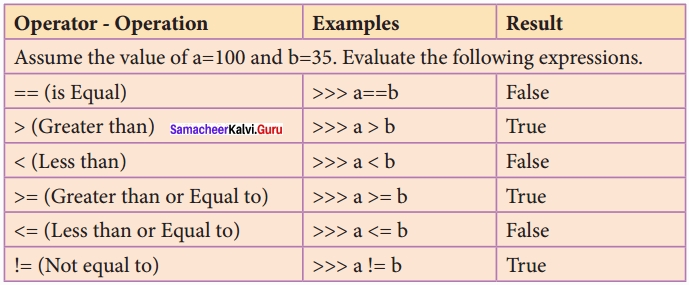Logical operators:
In python, Logical operators are used to performing logical operations on the given relational expressions. There are three logical operators they are and, or and not.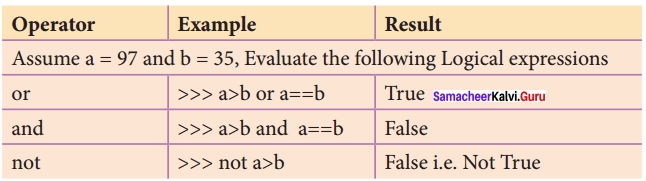Assignment operators:
In Python, = is a simple assignment operator to assign values to variables. Let a = 5 and b = 10 assigns the value 5 to a and 10 to b These two assignment statements can also be given as a, b = 5, 10 that assigns the value 5 and 10 on the right to the variables a and b respectively. There are various compound operators in Python like + =, – =, * =, / =, % =, ** = and //= are also available.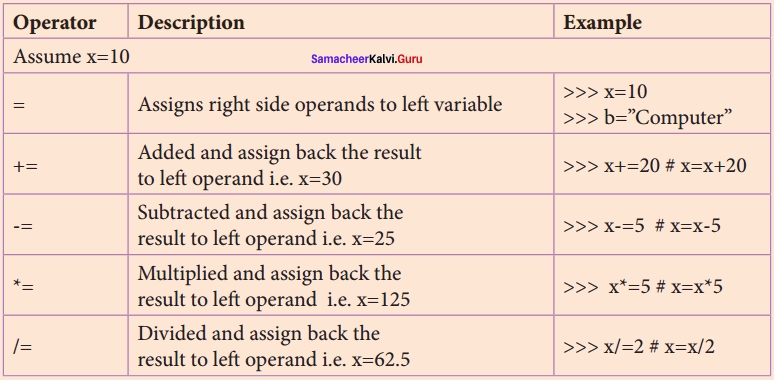Conditional operator:
A ternary operator is also known as a conditional operator that evaluates something based on a condition being true or false. It simply allows testing a condition in a single line replacing the multiline if-else making the code compact.
The Syntax conditional operator is,
Variable Name = [on _ true] if [Test expression] else [on _ false]
Example:
min = 50 if 49 < 50 else 70 # min = 50 min = 50 if 49 > 50 else 70 # min = 70

(IV) Delimiters
Python uses the symbols and symbol combinations as delimiters in expressions, lists, dictionaries and strings. Following are the delimiters.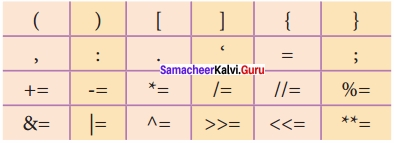(V) Literals
Literal is raw data given in a variable or constant. In Python, there are various types of literals.

1. Numeric
2. String
3. Boolean

1. Numeric Literals:
Numeric Literals consists of digits and are immutable (unchangeable). Numeric literals can belong to 3 different numerical types Integer, Float and Complex.

2. String Literals:
In Python a string literal is a sequence of characters surrounded by quotes. Python supports single, double and triple quotes for a string. A character literal is a single character surrounded by single or double-quotes. The value with triple-quote is used to give multi-line string literal.

3. Boolean Literals:
A Boolean literal can have any of the two values: True or False.

Escape Sequences:
In Python strings, the backslash “\” is a special character, also called the “escape” character. It is used in representing certain whitespace characters: “\t” is a tab, “\n” is a new line, and “\r” is a carriage return. For example to print the message “It’s raining”, the Python command is
>>>print (“It\’s raining”)
It’s raining
Python supports the following escape sequence characters.### Samacheer Kalvi 12th Computer Science Python – Variables and Operators Additional Questions and Answers

PART – 1

Question 1.
Python language was released in the year …………………………….
(a) 1991
(b) 1993
(c) 1995
(d) 1997
(a) 1991

Question 2.
IDLE expansion is
a) Information Development Logical Environment
b) Integrated Development Learning Environment
c) Integrated Development Language Environment
d) Interactive Development Learning Environment
b) Integrated Development Learning Environment

Question 3.
Python got its name from ……………………………
Monthly Python’s Flying Circus

Question 4.
In Python, the script mode programs can be stored with the extension.
a) .pyt
b) .py
c) .pyh
d) .pon
b) .py

Question 5.
IDLE means ……………………………
Integrated Development Learning Environment

Question 6.
How many modes of programming are there in python?
(a) 2
(b) 3
(c) 4
(d) 5
(a) 2

Question 7.
A sequence of elementary lexical components of Python statement is known as
a) Tokens
b) Keywords
c) Delimiters
d) Literals
a) Tokens

Question 8.
…………………………… mode is used to create and edit Python source file.
(a) Line
(b) Script
(c) Interactive
(d) Interface
(b) Script

Question 9.
Which mode can be used as a simple calculator?
(a) Line
(b) Script
(c) Interactive
(d) Interface
(c) Interactive

Question 10.
The ………….. command is used to open the Python shell window.
a) File → File New
b) File→ New
c) File → File Open
d) File → New File
d) File → New File

Question 11.
Which command is used to get output ……………………………
(a) Cout
(b) Print
(c) Print f
(d) Write
(b) Print

Question 12.
Find the correct one from the following.
(a) Scripts are reusable and not editable
(b) Scripts are not reusable and they are editable
(c) Scripts are not reusable, not editable
(d) Scripts are both reusable and editable
(d) Scripts are both reusable and editable

Question 13.
In Python, ……………..is a simple assignment operator
a) =
b) =
c) ==
d) #
b) =

Question 14.
What is the default name for a blank script text editor?
(a) Untitled
(b) Untitled 1
(c) Document 1
(d) Editor 1
(b) Untitled 1

Question 15.
What is the keyboard shortcut to Run?
(a) F5
(b) Alt + F5
(c) Shift + F5
(d) Ctrl + F5
(a) F5

Question 16.
A complex number is made up of two …………. values.
a) Integer
b) String
c) octal
d) floating-point
d) floating-point

Question 17.
Errors in the python script appear in …………………………… color.
(a) Yellow
(b) Red
(c) Blue
(d) Black
(b) Red

Question 18.
…………………………… function helps to enter data at run time by the user.
input ( )

Question 19.
If …………………………… is not given in input ( ), no message is displayed on the screen.
Prompt sting

Question 20.
Identify the wrong statement from the following. The input ( ) accepts all data as
(a) Strings
(b) Characters
(c) Numbers
(d) All the above
(c) Numbers

Question 21.
The …………………………… function is used to convert string data as integer data explicitly.
int ( )

Question 22.
…………………………… are ignored by the python interpreter.
(a) Keywords
(b) Tokens
(c) Delimiters

Question 23.
Comments are of …………………………… or …………………………… lines.
Single, multi

Question 24.
…………………………… are used to indicate blocks of codes in python.
(a) Whitespaces
(b) { }
(c) [ ]
(d) < >
(a) Whitespaces

Question 25.
Python breaks each logical line into a sequence of elementary lexical components called ……………………………
Tokens

Question 26.
How many types of tokens are there?
(a) 1
(b) 2
(c) 5
(d) 10
(c) 5

Question 27.
Pick the odd one out.

Question 28.
An identifier is a name used to identify a ……………………………
(a) Variable
(b) Function
(c) Class
(d) All of these
(d) All of these

Question 29.
Pick the odd one out.
(a) Sum, regno, numl, 12Name, – Marks
(b) False, class, is, as, if, end
(c) Relational, while, logical, Assignment,
(d) + * % ** = =
(a) 12 Name, (b) end, (c) while, (d) =

Question 30.
Find the correct statement from the following
(a) Continue is an identifier
(b) Sum is a keyword
(c) ** = is a delimiter
(d) = = is an assignment operator
(c) ** = is a delimiter

Question 31.
How many types of operators are there?
(a) 2
(b) 3
(c) 4
(d) 5
(d) 5

Question 32.
Match the following
1. // – (i) Modulus
2. # – (ii) Floor division
3. % – (iii) Strings
4. ||| ||| – (iv) Comments
(a) 1 – (ii), 2 – (iv), 3 – (i), 4 – (iii)
(b) 1 – (i), 2 – (ii), 3 – (iii), 4 – (iv)
(c) 1 – (iv), 2 – (ii), 3 – (i), 4 – (iii)
(d) 1 – (iv), 2 – (i), 3 – (iii), 4 – (ii)
(a) 1 – (ii), 2 – (iv), 3 – (i), 4 – (iii)

Question 33.
…………………………… are special words used by Python Interpreter
Keywords

Question 34.
The value of an operator used is ……………………………
(a) 0
(b) 1
(c) Operands
(d) NULL
(c) Operands

Question 37.
Match the following expressions with their equivalent output a = 100, b = 10
(1) >>> a % 30 – (i) 100
(2) >>> a // 30 – (ii) 10.0
(3) >>> a/b – (iii) 3
(4) >>> a * b – (iv) 10
(a) 1 – (iv), 2 – (iii), 3 – (ii), 4 – (i)
(b) 1 – (i), 2 – (ii), 3 – (iii), 4 – (iv)
(c) 1 – (i), 2 – (ii), 3 – (iv), 4 – (iii)
(d) 1 – (iv), 2 – (ii), 3 – (iii), 4 – (i)
(a) 1 – (iv), 2 – (iii), 3 – (ii), 4 – (i)

Question 38.
Which operator checks the relationship between two operands.
(a) Relational
(b) Comparative
(c) Both
(d) None of the these
(c) Both

Question 39.
Assume a = 100 and b = 35. Find the true statements.
(i) >>> a > b
(ii) >>> a = = b
(iii) >>> a ! = b
(a) (i), (iii) are true
(b) (ii), (iii) are true
(c) (i), (ii) are true
(a) (i), (iii) are true

Question 40.
Identify Not equal to the operator in python?
(a) < >
(b) ==
(c) NOT EQUAL
(d) ! =
(d) ! =

Question 41.
How many logical operators are there?
(a) 2
(b) 3
(c) 4
(d) 5
(b) 3

Question 42.
How many comparative operators are there?
(a) 4
(b) 5
(c) 6
(d) 7
(c) 6

Question 43.
…………………………… is the simple assignment operator.
(a) ! =
(b) >
(c) >>
(d) =
(d) =

Question 44.
Compound operators come under the category of …………………………… operators.
Assignment

Question 45.
Find the wrongly matched pair.
(a) ! = – relational operator
(b) not a > b – Comparative Operator
(c) **= – delimitors operator
(d) Assingnment Operator
(b) not a > b – Comparative Operator

Question 46.
Identify which is not a delimiter
(a) & =
(b) :
(c) ;
(d) ::
(d) ::

Question 47.
How many types of literals are there?
(a) 2
(b) 3
(c) 4
(d) 5
(b) 3

Question 48.
Numeric literals are ……………………………
(a) Integer, Float, Complex
(b) Int, Float, Void
(c) Int, Float, Char
(d) Int, Float, Boolean
(a) Integer, Float, Complex

Question 49.
Strings in python are represented using
(a) ”
(b) “”
(c) “‘ “‘
(d) All of these
(d) All of these

Question 50.
Multiline string literal is given by ……………………………
“‘ “‘ triple quotes

Question 51.
The two values accepted by Boolean literals are …………………………… or ……………………………
True, False

Question 52.
…………………………… is the escape character.
(a) +
(b) t
(c) \
(d) %
(c) \

Question 53.
Which one of the following is the newline character?
(a) \t
(b) \v
(c) \r
(d) \n
(d) \n

Question 54.
…………………………… is the escape character for carriage return.
\r

Question 55.
Pick the odd one out.
(a) Tuples, for, list, dictionaries, Number
(b) \n, \”, V, \r, \k
(c) and, or, not, true
(d) >, > =, <, < =, < >
(a) for, (b) \k, (c) true, (d) <>

Question 56.
How much Integer data are there?
(a) 2
(b) 3
(c) 4
(d) 5
(b) 3

Question 57.
OX represents …………………………… integer.

Question 58.
Octal integer uses …………………………… to denote octal digits
(a) OX
(b) O
(c) OC
(d) Od
(b) O

Question 59.
(a) 0102
(b) 0876
(c) 0432
(d) 0X102
(d) 0X102

Question 60.
How many floating-point values are there is a complex number?
(a) 1
(b) 2
(c) 3
(d) 4
(b) 2

Question 61.
What is another name for fundamental data type?
(a) Class
(b) Built – in
(c) Typedef
(d) User defined
(b) Built-in

Question 62.
Which one of the following statements is wrong?
(a) Octal Integer uses upper and lower case O
(b) Hexadecimal Integer uses upper and lower case OX
(c) Long integer uses upper and lower case 1.
(c) Long integer uses upper and lower case 1.

PART – II

Question 1.
How will you create scripts in python?
(I) Choose File ? New File or press Ctrl + N in Python shell window.(II) An untitled blank script text editor will be displayed on the screen.

Question 2.
What is the purpose of using Input-output functions?

• The input () function helps to enter data at run time by the user and the output function
• print () is used to display the result of the program on the screen after execution.

PART – III

Question 1.
What are the rules followed while defining the python identifier?

• An identifier must start with an alphabet (A..Z or a..z) or underscore (_)..
Identifiers may contain digits (0 .. 9).
• Python identifiers are case sensitive i.e. uppercase and lowercase letters are distinct.
• Identifiers must not be a python keyword.
• Python does not allow punctuation characters such as %,$, @, etc., within identifiers. Question 2. How will you execute the python script? Executing Python Script (I) Choose Run ? Run Module or Press F5(II) If your code has an error, it will be shown in red color in the IDLE window, and Python describes the type of error that occurred. To correct the errors, go back to the Script editor, make corrections, save the file using Ctrl + S or File ? Save and execute it again. (III) For all error-free code, the output will appear in the IDLE window of Python. Question 3. Give the syntax for the print ( ) function? Answer: The syntax for print ( ) is as follows: Example: print (“string to be displayed as output ” ) print (variable) print (“String to be displayed as output ”, variable) print (“String 1 ”, variable, “String 2”, variable, “String 3”) Question 4. Explain the Syntax of input ( ) function? Answer: The syntax for inputQ function is, Variable = input (“prompt string”) Where, prompt string in the syntax is a statement or message to the user, to know what input can be given. If a prompt string is used, it is displayed on the monitor; the user can provide expected data from the input device. The input) ) takes whatever is typed from the keyboard and stores the entered data in the given variable. If prompt string is not given in input() no message is displayed on the screen Question 5. Give an example for input with and without prompt string? Example 1: input ( ) with prompt string >>> city = input (“Enter Your City: ”) Enter Your City: Madurai Example 2: input ( ) without prompt string >>> city = input ( ) Rajarajan Question 6. Write a short note on comments? Answer: Comments in Python In Python, comments begin with hash symbol (#). The lines that begin with # are considered as comments and ignored by the Python interpreter. Comments may be single line or no multi-lines. The multiline comments should be enclosed within a set of # as given below. # It is Single line Comment # It is multiline comment which contains more than one line # Question 7. Mention some rules for Identifiers? Answer: 1. An identifier must start with an alphabet (A..Z or a..z) or underscore (_). 2. Identifiers may contain digits (0 .. 9) 3. Python identifiers are case sensitive i.e. uppercase and lowercase letters are distinct. 4. Identifiers must not be a python keyword. 5. Python does not allow punctuation character such as %,$, @ etc., within identifiers.

Question 8.
Give any 6 keywords in Python?
false, class, none, continue, finally, return, is

Question 9.
Give an example program for Ternary operator?
To test Conditional (Ternary), Operator:
# Program to demonstrate conditional operator
a, b = 30, 20
# Copy value of a in min if a < b else copy b
min = a if a < b else b print (“The Minimum of A and B is “, min) # End of the Program Output: The Minimum of A and B is 20.

Question 10.
Write any 6 delimiters in python?PART – IV

Question 1.
Explain operators in detail?
Operators: In computer programming languages operators are special symbols which represent computations, conditional matching, etc. The value of an operator used is called operands. Operators are categorized as Arithmetic, Relational, Logical, Assignment, etc. Value and variables when used with the operator are known as operands.

(I) Arithematic operators An arithmetic operator is a mathematical operator that takes two operands and performs a calculation on them. They are used for simple arithmetic. Most computer languages contain a set of such operators that can be used within equations to perform different types of sequential calculations.

Python supports the following Arithmetic operators.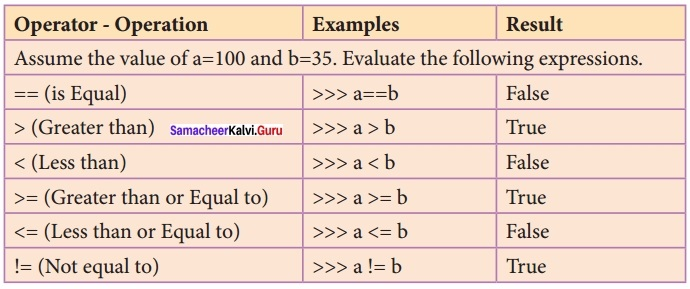To test Arithmetic Operators: #Demo Program to test Arithmetic Operators a = 100 b = 10 print (“The Sum = “,a + b) print (“The Difference = “,a – b) print (“The Product = “,a * b) print (“The Quotient = “,a / b) print (“The Remainder = “,a % 30) print (“The Exponent = “, a ** 2) print (“The Floor Division =”, a // 30) #Program End Output: The Sum = 110 The Difference = 90 The Product = 1000 The Quotient = 10.0 The Remainder = 10 The Exponent = 10000 The Floor Division = 3

(II) Relational or Comparative operators A Relational operator is also called a Comparative operator which checks the relationship between two operands. If the relation is true, it returns True; otherwise, it returns False.To test Relational Operators: #Demo Program to test Relational Operators a = int (input(“Enter a Value for A:”)) b = int (input(“Enter a Value for B:”)) print (“A = “,a,” and B = “,b) print (“The a = = b = “,a = = b) print (“The a > b = “,a > b)
print (“The a < b = “,a < b) print (“The a > = b = “, a > = b)
print (“The a! = b = “,a! = 0)
print (“The a! = b = “,a! = b)
#Program End

Output:
Enter a Value for A: 35
Enter a Value for B: 56
A = 35 and B = 56
The a = =b = False
The a > b = False
The a < b = True The a > = b = False
The a < = b = False
The a ! = b = True

(III) Logical operators
In python, Logical operators are used to performing logical operations on the given relational expressions. There are three logical operators they are and, or and not.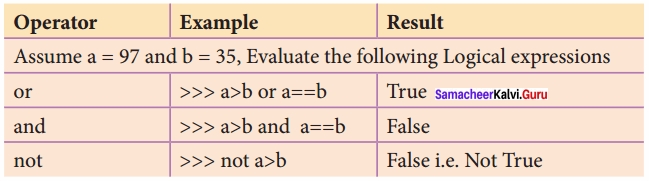To test Logical Operators:(IV) Assignment operators
In Python, = is a simple assignment operator to assign values to variables. Let a = 5 and b = 10 assigns the value 5 to a and 10 to b this two assignment statement can also be given as a, b = 5, 10 that assigns the value 5 and 10 on the right to the variables a and b respectively. There are various compound operators in Python like + =, – =, * =, /=, % =, ** = and //= are also available.To test Assignment Operators:(V) Conditional operator
A ternary operator is also known as a conditional operator that evaluates something based on a condition being true or false. It simply allows testing a condition in a single line replacing the multiline if-else making the code compact.
The Syntax conditional operator is,
Variable Name = [on _ true] if [Test expression] else [on _ false]
Example:
min = 50 if 49 < 50 else 70 # min = 50 min = 50 if 49 > 50 else 70 # min = 70
To test Conditional (Ternary) Operator:
# Program to demonstrate conditional operator
a, b = 30, 20
# Copy value of a in min if a < b else copy b
min = a if a < b else b print (“The Minimum of A and B is “,min) # End of the Program Output: The Minimum of A and B is 20.

Question 2.
Write the Output for the given program?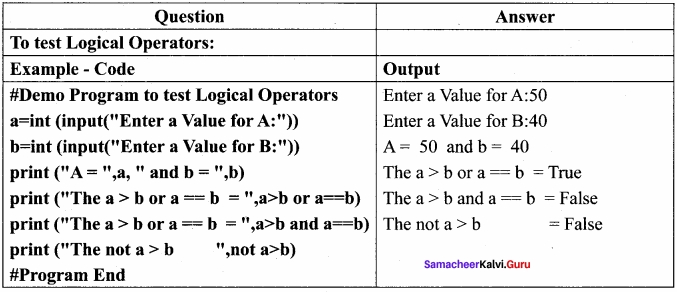Question 3.
Explain literals and their types?
Literal is raw data given in a variable or constant. In Python, there are various types of literals.

(I) Numeric Literals Numeric Literals consists of digits and are immutable (unchangeable). Numeric literals can belong to 3 different numerical types Integer, Float and Complex. To demonstrate Numeric literals # Program to demonstrate Numeric Literals a = Ob 1010 #Binary Literals b = 100 #Decimal Literal c = 0o310 #Octal Literal d = Ox 12c #Hexadecimal Literal print (“Integer Literals :”,a, b, c, d) #Float Literal float_1 = 10.5 float_2 = 1.5e2 print (“Float Literals :”,float_1, float_2) #Complex Literal x = 1 + 3.14 j print (“Complex Literals:”, x) Print (“x = “, x , “Imaginary part of x = “, x.imag, “Real part of x = “, x.real) #End of the Program Output: Integer Literals: 10 100 200 300 Float Literals: 10.5 150.0 Complex Literals: x = (1.3.14) Imaginary part of x = 3.14 Real part of 9 x = 1.0

(II) String Literals: In Python, a string literal is a sequence of characters surrounded by quotes. Python supports single, double, and triple quotes for a string. A character literal is a single character surrounded by single or double-quotes. The value with triple-quote is used to give multi-line string literal. To test String Literals # Demo Program to test String Literals strings = “This is Python” char = “C” multiline_str = ‘This is a multiline string with more than one line code.’ print (strings) print (char) print (multiline_str) # End of the Program Output: This is Python C C This is a multiline string with more than one line code.

(III) Boolean Literals A Boolean literal can have any of the two values: True or False. # Demo Program to test String Literals boolean _ 1 = True boolean _ 2 = False print (“Demo Program for Boolean Literals”) print (“Boolean Value 1 :”,boolean_1) print (“Boolean Value2 :”,boolean_2) # End of the Program Output: Demo Program for Boolean Literals Boolean Value 1: True Boolean Value2: False (iv) Escape Sequences In Python strings, the backslash “\” is a special character, also called the “escape” character. It is used in representing certain whitespace characters: “\t” is a tab, “\n” is a new line, and “\r” is a carriage return. For example to print the message “It’s raining”, the Python command is >>> print (“ItVs raining”)
It’s raining
Python supports the following escape sequence characters.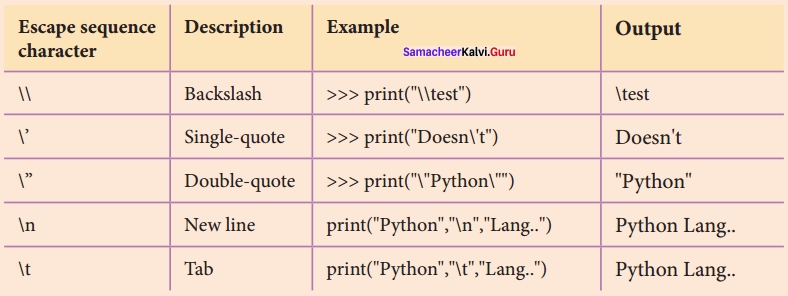Question 4.
Explain the key features and coding of Python.